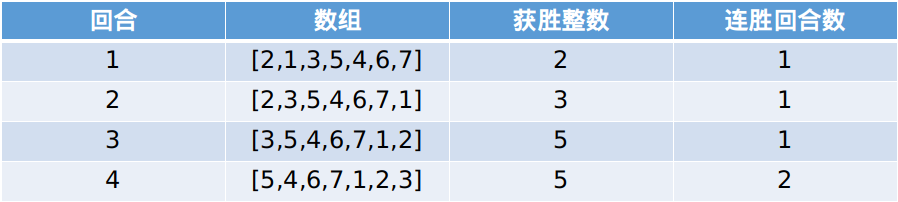# 找出数组游戏的赢家

## 1535. 找出数组游戏的赢家 (Medium)

```输入：arr = [2,1,3,5,4,6,7], k = 2```

```输入：arr = [3,2,1], k = 10

```

```输入：arr = [1,9,8,2,3,7,6,4,5], k = 7

```

```输入：arr = [1,11,22,33,44,55,66,77,88,99], k = 1000000000

```

• `2 <= arr.length <= 10^5`
• `1 <= arr[i] <= 10^6`
• `arr` 所含的整数 各不相同
• `1 <= k <= 10^9`

[数组] [模拟]